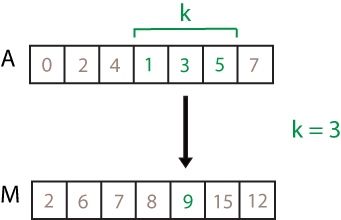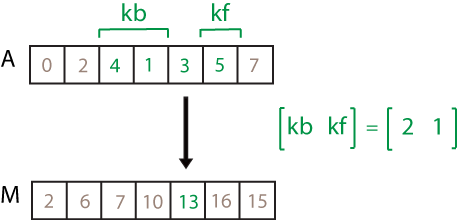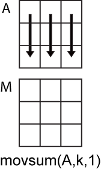Documentation

movsum

Description

example

M = movsum(A,k) returns an array of local k-point sums, where each sum is calculated over a sliding window of length k across neighboring elements of A. When k is odd, the window is centered about the element in the current position. When k is even, the window is centered about the current and previous elements. The window size is automatically truncated at the endpoints when there are not enough elements to fill the window. When the window is truncated, the sum is taken over only the elements that fill the window. M is the same size as A.

• If A is a vector, then movsum operates along the length of the vector.

• If A is a multidimensional array, then movsum operates along the first array dimension whose size does not equal 1.

example

M = movsum(A,[kb kf]) computes the sum with a window of length kb+kf+1 that includes the element in the current position, kb elements backward, and kf elements forward.

example

M = movsum(___,dim) returns the array of sliding sums along dimension dim for any of the previous syntaxes. For example, if A is a matrix, then movsum(A,k,2) operates along the columns of A, computing the k-element sliding sum for each row.

example

M = movsum(___,nanflag) specifies whether to include or omit NaN values from the calculation for any of the previous syntaxes. movsum(A,k,'includenan') includes all NaN values in the calculation while movsum(A,k,'omitnan') ignores them and computes the sum over fewer points.

example

M = movsum(___,Name,Value) specifies additional parameters for the sum using one or more name-value pair arguments. For example, if x is a time vector, then movsum(A,k,'SamplePoints',x) computes the moving sum of A relative to the times in x.

Examples

collapse all

Compute the three-point centered moving sum of a row vector. When there are fewer than three elements in the window at the endpoints, take the sum over the elements that are available.

A = [4 8 6 -1 -2 -3 -1 3 4 5];
M = movsum(A,3)
M = 1×10

12    18    13     3    -6    -6    -1     6    12     9

Compute the three-point trailing moving sum of a row vector. When there are fewer than three elements in the window at the endpoints, movsum takes the sum over the number of elements that are available.

A = [4 8 6 -1 -2 -3 -1 3 4 5];
M = movsum(A,[2 0])
M = 1×10

4    12    18    13     3    -6    -6    -1     6    12

Compute the three-point centered moving sum for each row of a matrix. The window starts on the first row, slides horizontally to the end of the row, then moves to the second row, and so on. The dimension argument is two, which slides the window across the columns of A.

A = [4 8 6; -1 -2 -3; -1 3 4]
A = 3×3

4     8     6
-1    -2    -3
-1     3     4

M = movsum(A,3,2)
M = 3×3

12    18    14
-3    -6    -5
2     6     7

Compute the three-point centered moving sum of a row vector containing two NaN elements.

A = [4 8 NaN -1 -2 -3 NaN 3 4 5];
M = movsum(A,3)
M = 1×10

12   NaN   NaN   NaN    -6   NaN   NaN   NaN    12     9

Recalculate the sum, but omit the NaN values. When movsum discards NaN elements, it takes the sum over the remaining elements in the window.

M = movsum(A,3,'omitnan')
M = 1×10

12    12     7    -3    -6    -5     0     7    12     9

Compute a 3-hour centered moving sum of the data in A according to the time vector t.

A = [4 8 6 -1 -2 -3];
k = hours(3);
t = datetime(16,1,1,0,0,0) + hours(0:5)
t = 1x6 datetime array
Columns 1 through 3

01-Jan-0016 00:00:00   01-Jan-0016 01:00:00   01-Jan-0016 02:00:00

Columns 4 through 6

01-Jan-0016 03:00:00   01-Jan-0016 04:00:00   01-Jan-0016 05:00:00

M = movsum(A,k,'SamplePoints',t)
M = 1×6

12    18    13     3    -6    -5

Compute the three-point centered moving sum of a row vector, but discard any calculation that uses fewer than three points from the output. In other words, return only the sums computed from a full three-element window, discarding endpoint calculations.

A = [4 8 6 -1 -2 -3 -1 3 4 5];
M = 1×8

18    13     3    -6    -6    -1     6    12

Input Arguments

collapse all

Input array, specified as a vector, matrix, or multidimensional array.

Data Types: single | double | int8 | int16 | int32 | int64 | uint8 | uint16 | uint32 | uint64 | logical

Window length, specified as a numeric or duration scalar. When k is a positive integer scalar, the centered sum includes the element in the current position plus surrounding neighbors. For example, a three-point sum defined by a window of length three results in the following calculation for a vector A:Data Types: single | double | int8 | int16 | int32 | int64 | uint8 | uint16 | uint32 | uint64 | duration

Directional window length, specified as a numeric or duration row vector containing two elements. When kb and kf are positive integer scalars, the calculation is over kb+kf+1 elements. The calculation includes the element in the current position, kb elements before the current position, and kf elements after the current position. For example, a four-point sum defined by the directional window [2 1] results in the following calculation for a vector A:Data Types: single | double | int8 | int16 | int32 | int64 | uint8 | uint16 | uint32 | uint64 | duration

Dimension to operate along, specified as a positive integer scalar. If no value is specified, then the default is the first array dimension whose size does not equal 1.

Dimension dim indicates the dimension that movsum operates along, that is, the direction in which the specified window slides.

Consider a two-dimensional input array, A.

• If dim = 1, then movsum(A,k,1) starts with the first column and slides vertically over each row. The sum is computed over k elements at a time. Then it moves to the second column and repeats the computation. This process continues until all columns are exhausted.• If dim = 2, then movsum(A,k,2) starts with the first row and slides horizontally across each column. The sum is computed over k elements at a time. Then it moves to the second row and repeats the computation. This process continues until all rows are exhausted.Data Types: double | single | int8 | int16 | int32 | int64 | uint8 | uint16 | uint32 | uint64

NaN condition, specified as one of these values:

• 'includenan' — Include NaN values when computing the sum, resulting in NaN.

• 'omitnan' — Ignore all NaN values in the input. If a window contains only NaN values, then movsum returns 0.

Name-Value Pair Arguments

Specify optional comma-separated pairs of Name,Value arguments. Name is the argument name and Value is the corresponding value. Name must appear inside quotes. You can specify several name and value pair arguments in any order as Name1,Value1,...,NameN,ValueN.

Example: M = movsum(A,k,'Endpoints','fill')

Method to treat leading and trailing windows, specified as the comma-separated pair consisting of 'Endpoints' and one of the following:

'Endpoints' ValueDescription
'shrink'Shrink the window size near the endpoints of the input to include only existing elements.
'discard'Do not output any sums when the window does not completely overlap with existing elements.
'fill'Substitute nonexisting elements with NaN.
numeric or logical scalarSubstitute nonexisting elements with a specified numeric or logical value.

Data Types: double | single | int8 | int16 | int32 | int64 | uint8 | uint16 | uint32 | uint64 | logical | char | string

Sample points for computing sums, specified as the comma-separated pair consisting of 'SamplePoints' and a vector. The sample points represent the location of the data in A. Sample points do not need to be uniformly sampled. By default, the sample points vector is [1 2 3 ... ].

Moving windows are defined relative to the sample points, which must be sorted and contain unique elements. For example, if t is a vector of times corresponding to the input data, then movsum(rand(1,10),3,'SamplePoints',t) has a window that represents the time interval between t(i)-1.5 and t(i)+1.5.

When the sample points vector has data type datetime or duration, then the moving window length must have type duration.

If the sample points are nonuniformly spaced and the 'Endpoints' name-value pair is specified, then its value must be 'shrink'.

Data Types: single | double | int8 | int16 | int32 | int64 | uint8 | uint16 | uint32 | uint64 | datetime | duration